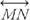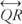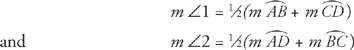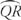## Other Angles

Theorem 73: If a tangent and a diameter meet at the point of tangency, then they are perpendicular to one another.

In Figure 1, diameter AB meets tangentat B. According to Theorem 73AB ⊥which means that m ∠ ABC = 90° and m ∠ ABD = 90°.Figure 1 A tangent to the circle and a diameter of the circle meeting at the point of tangency.

Theorem 74: If a chord is perpendicular to a tangent at the point of tangency, then it is a diameter.

Example 1: Theorem 74 could be used to find the center of a circle if two tangents to the circle were known. In Figure 2is tangent to the circle at Pis tangent to the circle at S. Use these facts to find the center of the circle.Figure 2 Finding the center of a circle when two tangents to the circle are known.

According to Theorem 74, if a chord is drawn perpendicular toat P, it is a diameter, which means that it passes through the center of the circle.

Similarly, if a chord is drawn perpendicular toat S, it too would be a diameter and pass through the center of the circle. The point where these two chords intersect would then be the center of the circle. See Figure 3.Figure 3 Chords drawn perpendicular to tangents to help in finding the center of the circle.

Theorem 75: The measure of an angle formed by two chords intersecting inside a circle is equal to half the sum of the measures of the intercepted arcs associated with the angle and its vertical angle counterpart.

In Figure 4, chords AC and BD intersect inside the circle at E.Figure 4 Angles formed by two chords intersecting inside a circle.

By Theorem 75:,Theorem 76: The measure of an angle formed by a tangent and a chord meeting at the point of tangency is half the measure of the intercepted arc.

In Figure 5, chord QR and tangentmeet at R. By Theorem 76m ∠1 = 1/2 ( m) and m ∠ 2 = ½ ( m).Figure 5 A tangent to the circle and a chord meeting at the point of tangency

Theorem 77: The measure of an angle formed by two secants intersecting outside a circle is equal to one half the difference of the measures of the intercepted arcs.

In Figure , secantsandintersect at G. According to Theorem 77m ∠1 = 1/2( m– m).Figure 6 Two secants to the circle meeting outside the circle.

Example 2: Find m ∠1 in Figures 7 (a) through (d).Figure 7 Angles formed by intersecting chords, secants, and/or tangents.

Example 3: Find the value of y in Figures 8 (a) through (d).Figure 8 Angles formed by intersecting chords, secants, and/or tangents.

Back to Top
A18ACD436D5A3997E3DA2573E3FD792A